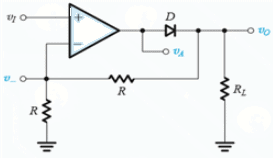Courses

# Test: Rectifiers

## 10 Questions MCQ Test Electronic Devices | Test: Rectifiers

Description
This mock test of Test: Rectifiers for Electrical Engineering (EE) helps you for every Electrical Engineering (EE) entrance exam. This contains 10 Multiple Choice Questions for Electrical Engineering (EE) Test: Rectifiers (mcq) to study with solutions a complete question bank. The solved questions answers in this Test: Rectifiers quiz give you a good mix of easy questions and tough questions. Electrical Engineering (EE) students definitely take this Test: Rectifiers exercise for a better result in the exam. You can find other Test: Rectifiers extra questions, long questions & short questions for Electrical Engineering (EE) on EduRev as well by searching above.
QUESTION: 1

### Which of the following isn’t a type of rectifier?

Solution:

All of the mentioned are different types of a rectifier.

QUESTION: 2

### For a half wave or full wave rectifier the Peak Inverse Voltage of the rectifier is always

Solution:

The peak input voltage is smaller than the input voltage due to the presence of diode(s). A single diode reduces the output voltage by approximately 0.7V.

QUESTION: 3

### For a half-wave rectifier having diode voltage VD and supply input of VI, the diode conducts for π – 2Θ, where Θ is given by

Solution:

The diode doesn’t conducts when VD ≥VI . Hence Θ = sin-1 (D/VI).

QUESTION: 4

Bridge rectifier is an alternative for

Solution:

Bridge rectifier is a better alternative for a full wave rectifier.

QUESTION: 5

Which of the following is true for a bridge rectifier?

Solution:

All of the given statements are true for a bridge rectifier.

QUESTION: 6

The diode rectifier works well enough if the supply voltage is much than greater than 0.7V. For smaller voltage (of few hundreds of millivolt) input which of the following can be used?

Solution:

For the supply voltages less than 0.7V super diodes are used.

QUESTION: 7

A simple diode rectifier has ‘ripples’ in the output wave which makes it unsuitable as a DC source. To overcome this one can use

Solution:

A capacitor is parallel with a resistor can only makes ripples go away. Series connection will become equal to an open circuit once the capacitor is fully charged.

QUESTION: 8

Consider a peak rectifier fed by a 60-Hz sinusoid having a peak value Vp = 100 V. Let the load resistance R = 10 kΩ. Calculate the fraction of the cycle during which the diode is conducting

Solution:

w Δt ~ √(2Vr/Vp)
Θ = √(2 X 2/100)
Θ = 0.2 rad or 3.18% of the cycle

QUESTION: 9

(Q.9-Q.10) The op amp in the precision rectifier circuit is ideal with output saturation levels of ±12 V. Assume that when conducting the diode exhibits a constant voltage drop of 0.7 V.Find V when VI is -1V.

Solution:

VI = -1v
Vo = 1v
VA = 1.7v
V = 0v
Virtual gnd as negative feedback is closed through R.
VI > 0 D1 conducts D2 cutoff.
VI < 0 D2 conducts D1 cutoff.
V0 ⁄ VI = -1.

QUESTION: 10

Find V0 when VI is 2V.

Solution:

: VI = 2v
Vo = 0v
VA = -0.7v
V = 0v
Virtual gnd as negative feedback is closed through R.
VI > 0 D1 conducts D2 cutoff.
VI < 0 D2 conducts D1 cutoff.
V0 ⁄ VI = -1.